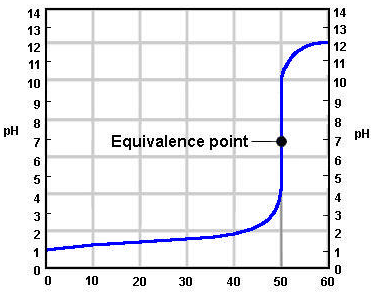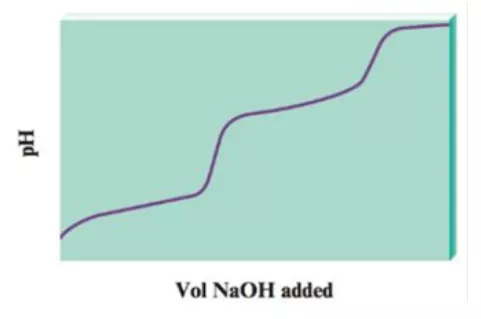###### FREE Expert Solution

a. Notice that the plot has essentially two titration curves. If the first equivalence point occurs at 100.0 mL NaOH added, what volume of NaOH added corresponds to the second equivalence point?

The equivalence point is located at the center of the high slope part of the curve.96% (169 ratings)###### Problem Details

When a diprotic acid, H2A, is titrated with NaOH, the protons on the diprotic acid are generally removed one at a time, resulting in a pH curve that has the following generic shape:a. Notice that the plot has essentially two titration curves. If the first equivalence point occurs at 100.0 mL NaOH added, what volume of NaOH added corresponds to the second equivalence point?

b. For the following volumes of NaOH added, list the major species present after OH- reacts completely.

ii. between 0 and 100 mL of NaOH added

iv. between 100 and 200 mL NaOH added